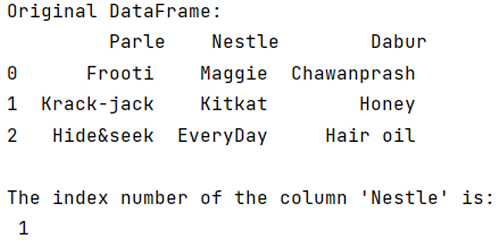# Get column index from column name in Python pandas

Given a DataFrame, we have to get column index from column name. By Pranit Sharma Last updated : September 19, 2023

Pandas is a special tool that allows us to perform complex manipulations of data effectively and efficiently. In a DataFrame, both rows and columns are assigned with an index value ranging from 0 to n-1. 0th is the first row and n-1th index is the last row. On the other hand, columns are the different fields that contain their particular values when we create a DataFrame. We can perform certain operations on both rows & column values. Here, we are going to learn how to get the index number of a column.

## Problem statement

Given a DataFrame, we have to get column index from column name.

## Getting pandas column index from column name

Pandas allow us to achieve this task using df.columns.get_loc() method. This method takes the name of the column as a parameter and returns the corresponding index number.

Note

To work with pandas, we need to import pandas package first, below is the syntax:

```import pandas as pd
```

Let us understand with the help of an example:

## Python program to get pandas column index from column name

```# Importing pandas package
import pandas as pd

# Defining a DataFrames
df = pd.DataFrame(data = {'Parle':['Frooti','Krack-jack','Hide&seek'],
'Nestle':['Maggie','Kitkat','EveryDay'],
'Dabur':['Chawanprash','Honey','Hair oil']})

# Display DataFrame
print("Original DataFrame:\n",df,"\n")

# Getting index number of "Nestle" column
result = df.columns.get_loc('Nestle')

# Display Result
print("The index number of the column 'Nestle' is:\n",result)
```

### Output

The output of the above program is: